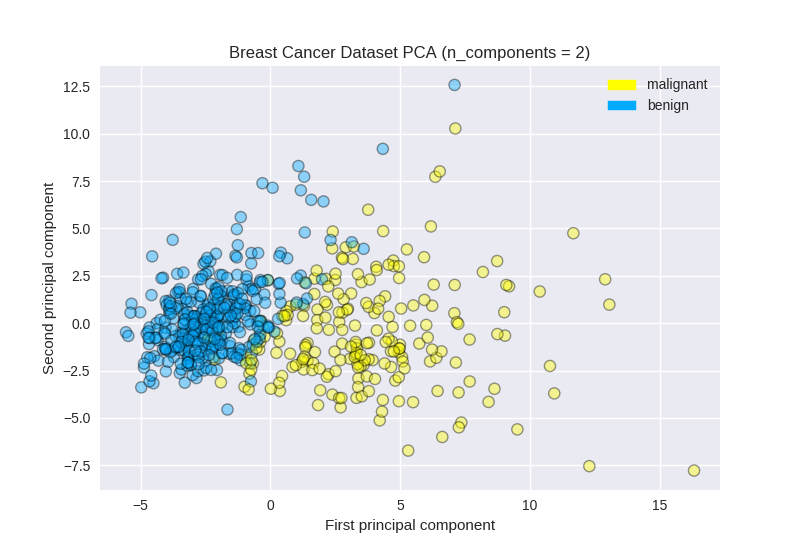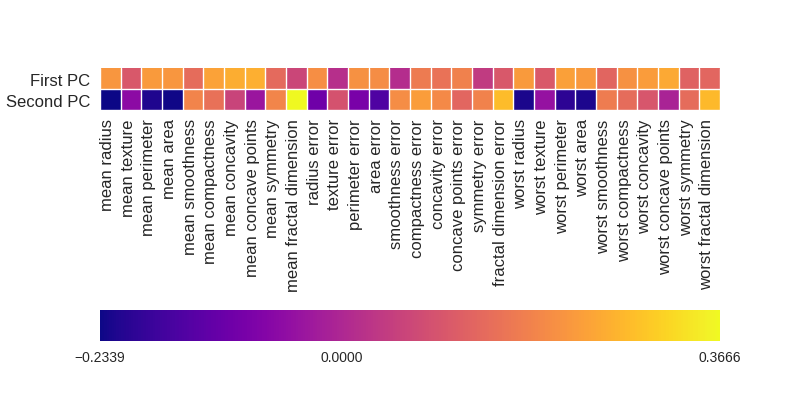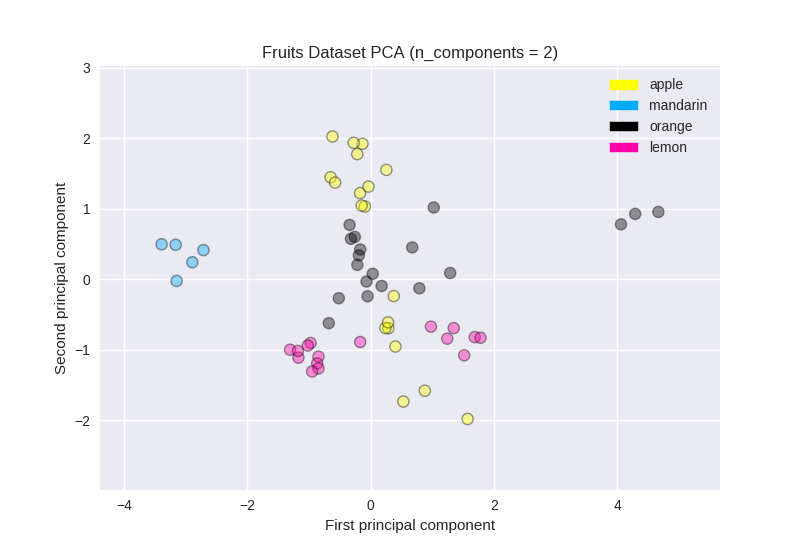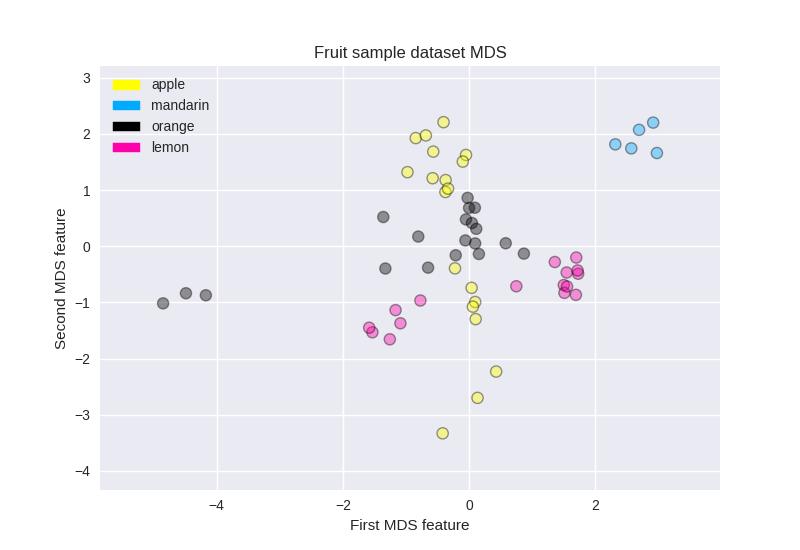# Applied Machine Learning: Unsupervised Learning¶

• Unsupervised learning involves tasks that operate on datasets without labeled repsonses or target values
• Instead, the goal is to capture interesting structure or information

Applications of unsupervised learning:

• Visualize structure of a complex dataset
• Density estimation to predict probabilities of events
• Compress and summarize the data
• Extract features for supervised learning
• Discover important clusters or outliers

Two major types of unsupervised learning methods:

• Transformations
• Processes that extract or compute information
• Clustering
• Find groups in the data
• Assign every point in the dataset to one of the groups

## Preamble and Datasets¶

In :
%matplotlib notebook
import numpy as np
import pandas as pd
import seaborn as sn
import matplotlib.pyplot as plt
from sklearn.datasets import load_breast_cancer

# Breast cancer dataset
(X_cancer, y_cancer) = load_breast_cancer(return_X_y = True)

# Our sample fruits dataset
X_fruits = fruits[['mass','width','height', 'color_score']]
y_fruits = fruits[['fruit_label']] - 1


## Dimensionality Reduction and Manifold Learning¶

The transformation category are known as dimensionality reduction algorithms. One every common need for dimensionality reduction arises when first exploring a dataset to understand how the samples may be grouped or related to each other by visualizing it using a two-dimensional scatterplot. One very important form of dimensionality reduction is called principal component analysis (PCA). Intuitively, what PCA does is take your cloud of original data points and finds a rotation of it, so the dimensions are statistically uncorrelated, PCA then typically drops all but the most informative initial dimensions that capture most of the variation in the original datset.

### Principal Components Analysis (PCA)¶

#### Using PCA to find the first two principal components of the breast cancer dataset¶

In :
from sklearn.preprocessing import StandardScaler
from sklearn.decomposition import PCA
from sklearn.datasets import load_breast_cancer

(X_cancer, y_cancer) = load_breast_cancer(return_X_y = True)

# Before applying PCA, each feature should be centered (zero mean) and with unit variance
X_normalized = StandardScaler().fit(X_cancer).transform(X_cancer)

pca = PCA(n_components = 2).fit(X_normalized)

X_pca = pca.transform(X_normalized)
print(X_cancer.shape, X_pca.shape)

(569, 30) (569, 2)


#### Plotting the PCA-transformed version of the breast cancer dataset¶

In :
from adspy_shared_utilities import plot_labelled_scatter
plot_labelled_scatter(X_pca, y_cancer, ['malignant', 'benign'])

plt.xlabel('First principal component')
plt.ylabel('Second principal component')
plt.title('Breast Cancer Dataset PCA (n_components = 2)');#### Plotting the magnitude of each feature value for the first two principal components¶

In :
fig = plt.figure(figsize=(8, 4))
plt.imshow(pca.components_, interpolation = 'none', cmap = 'plasma')
feature_names = list(cancer.feature_names)

plt.gca().set_xticks(np.arange(-.5, len(feature_names)));
plt.gca().set_yticks(np.arange(0.5, 2));
plt.gca().set_xticklabels(feature_names, rotation=90, ha='left', fontsize=12);
plt.gca().set_yticklabels(['First PC', 'Second PC'], va='bottom', fontsize=12);

plt.colorbar(orientation='horizontal', ticks=[pca.components_.min(), 0,#### PCA on the fruit dataset (for comparison)¶

In :
from sklearn.preprocessing import StandardScaler
from sklearn.decomposition import PCA

# each feature should be centered (zero mean) and with unit variance
X_normalized = StandardScaler().fit(X_fruits).transform(X_fruits)

pca = PCA(n_components = 2).fit(X_normalized)
X_pca = pca.transform(X_normalized)

from adspy_shared_utilities import plot_labelled_scatter
plot_labelled_scatter(X_pca, y_fruits, ['apple','mandarin','orange','lemon'])

plt.xlabel('First principal component')
plt.ylabel('Second principal component')
plt.title('Fruits Dataset PCA (n_components = 2)');### Manifold learning methods¶

#### Multidimensional scaling (MDS) on the fruit dataset¶

In :
from adspy_shared_utilities import plot_labelled_scatter
from sklearn.preprocessing import StandardScaler
from sklearn.manifold import MDS

# each feature should be centered (zero mean) and with unit variance
X_fruits_normalized = StandardScaler().fit(X_fruits).transform(X_fruits)

mds = MDS(n_components = 2)

X_fruits_mds = mds.fit_transform(X_fruits_normalized)

plot_labelled_scatter(X_fruits_mds, y_fruits, ['apple', 'mandarin', 'orange', 'lemon'])
plt.xlabel('First MDS feature')
plt.ylabel('Second MDS feature')
plt.title('Fruit sample dataset MDS');#### Multidimensional scaling (MDS) on the breast cancer dataset¶

(This example is not covered in the lecture video, but is included here so you can compare it to the results from PCA.)

In :
from sklearn.preprocessing import StandardScaler
from sklearn.manifold import MDS
from sklearn.datasets import load_breast_cancer

(X_cancer, y_cancer) = load_breast_cancer(return_X_y = True)

# each feature should be centered (zero mean) and with unit variance
X_normalized = StandardScaler().fit(X_cancer).transform(X_cancer)

mds = MDS(n_components = 2)

X_mds = mds.fit_transform(X_normalized)

from adspy_shared_utilities import plot_labelled_scatter
plot_labelled_scatter(X_mds, y_cancer, ['malignant', 'benign'])

plt.xlabel('First MDS dimension')
plt.ylabel('Second MDS dimension')
plt.title('Breast Cancer Dataset MDS (n_components = 2)');